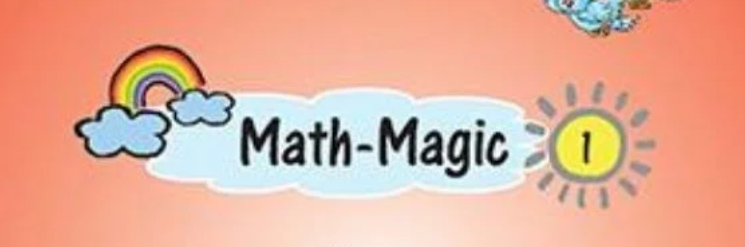NCERT Solutions for Class 1 Maths are given for the students so that they can get to know the answers to the questions in case they are not able to find it. It is important for all the students who are in Class 1 currently. Here we are providing the solutions to all the chapters of the NCERT Maths Class 1 Textbook for the students. They can refer to these solutions while they are solving the questions from the textbook.

## NCERT Solutions for Class 1 Maths

The students who are in class 1 and have the NCERT affiliated Maths Books can check this page to know the solutions for all the chapters of the Maths book. They need to ensure that they are checking the solutions for the chapter which they intend to check.

## NCERT Solutions for Class 1 Maths Book

NCERT Solutions for Class 1 Maths are given below for all chapters. Select chapter number to view NCERT Solution chapter wise

### Importance of the Solutions

The NCERT Solutions for Class 1 Maths are important for the students of the same class. Through these, they can get answers to those questions on which they get stuck. These are the valid solutions and students can check these whenever they face any confusion in the questions.

### NCERT Solutions Subject wise

We are giving the subject wise solution of NCERT Class 1 books. They will find chapter wise solutions for each subject which can be downloaded.